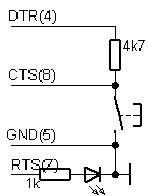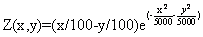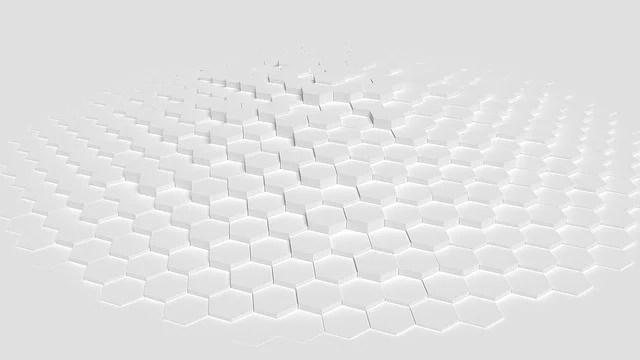## Controlling external devices using COM port communications programmed using VB languageThere are a lot of Radio amateurs that want to control external devices using computer standard ports. One of them is COM poert. Everybody wants things to be ease as people doing electronics are more hardware people not software. COM port is more often used than LPT because COM port is more resistive to bigger loads and there is less chances of failing. So if you know Visual Basic a little bit then this shouldn’t be very hard to use MSComm Control component which is located in Project->Components. You should check check box MSComm Control. Later you have to add this control to form and write some code for it. Main difficulty with this is that you have to follow RS232 protocol. This is why it is better to use microcontrollers that have built in USART interface. Of course MSComm component allows to read and control single COM pins and this way to control any external devices without using RS232 protocol. One good example is popular programming software PonyProg (which is programmed in other language than VB, but principals are same). You can see various supported circuits that PonyProg supports and you can see that Rx(2) and Tx(3) signals aren’t…

## Sample routine of working with LPT1 port under windows XP in CPP languageThis is simple routine of sending and reading of byte from LPT1 port under Windows XP. LPT port has four types of pins: 8 output pins accessed via the DATA Port 5 input pins (one inverted) accessed via the STATUS Port 4 output pins (three inverted) accessed via the CONTROL Port The remaining 8 pins are grounded Now we are interested in Data pins. Set up driver according to post: Acces LPT and COM ports easily under windows NT-2000-XP. I have written and compiled example under DEV-CPP tool-set, which you can download from https://www.bloodshed.net/. Start New console project Create new cpp file and save it to project directory. Also copy porttalk_IOCTL.h and pt_ioctl.c files to project directory. These files you will find in the package portalk22.zip. Test program: Compile this program and run it. You should see results like this: Now it is time to connect your microcontroller and start experimenting. Good luck. Test routine project files for DEV-CPP are here:LPT1 Sample Project

## Program LPT and COM ports easily under windows NT-2000-XP## Variables in embedded C programming languageWhat are variables in C language. Variables are simple keywords which are defined by the values. Values can be changed. Variables are like a boxes with some size where values like apples can be put in. So variables can be various forms and sizes so called variable types. Variable type is defined by a reserved word which indicates the type and size of variable identifier: unsigned char my_char; long int all_my_numbers; int number; Why do we need variables? The basic answer is that memory is limited and compiler needs to know much space to reserve for each variable. The coder needs to specify the variable type and its size by using one of reserved words from the table:

## Gauss-Zeidel optimization routinesThis is simplest optimization routines. Using this algorithm optimization parameters are changed separately in each step. Only one parameter can be changed in one step while other are helt as constants. Xk+1=Xk+ΔXk , k=0,1,2,… ΔXk step of parameter Xk. Parameter is changed until function growth is noticed, and then next parameter follows and so on. After cycle with all parameters is completed, then step is changed to half of its value and repeat cycle again. Optimal point searching ends when there is no function increase and last point is held as optimal point. Lets see how it works with following function: Its plot: Using MATLAB script we get results bellow. In each picture start coordinates are different. Start coordinates. x=150; y=200; Start coordinates x=50; y=150; Other example Start coordinates x=10; y=10; Start coordinates x=100; y=200; Third example Start coordinates x=10; y=10; Start coordinates x=50; y=200; Matlab script: close all; clear all; clc; [X,Y] = meshgrid(-100:1:100, -100:1:100); Z =3*exp(-((X.^2)/78000) -((Y.^2)/20000))-5*exp(-(((X+31).^2)/123) -(((Y+20).^2)/5000)); xx=100; yy=100; contour3(Z,20); hold on; % figure(2); mesh(Z); % figure % plot3(X,Y,Z) X=70; Y=100; X1=0;Y1=0; X0=X; Y0=Y; z=160; figure(1); plot(X,Y,’r*’), hold on; X=X-xx; Y=Y-yy; TT1 =3*exp(-((X.^2)/78000) -((Y.^2)/20000))-5*exp(-(((X+31).^2)/123) -(((Y+20).^2)/5053)); for i=1:50 z=z/2; X=X-z; Z2 =3*exp(-((X.^2)/78000) -((Y.^2)/20000))-5*exp(-(((X+31).^2)/123) -(((Y+20).^2)/5053)); if Z2>=TT1 TT=Z2; X1=X;…

## Impulse Response of discrete systemImpulse signal can be represented as: d[n] = 1, if n=0 d[n] = 0, otherwise it can also be written like d=[1,0,0,0,â€¦] Impulse Response The impulse response h(n) is the response of filter L() at time n to unit impulse occurring at time 0. h(n)=L(d(n)) Lets see how discrete system can be described when impulse response is known We know that: In the linear system this can be written as follows: Because h(n-k)=L(d(n-k)) Then: What do we get? There is obvious, that linear system can be described by its impulse response. The last expression is called convolution. This is the heart of DSP Filtering. To write this sum in more convenient matter is assumed that: Matlab example Matlab example: % Plot an unit impulse signal n = -7:7; x = [0 0 0 0 0 0 0 1 2 3 0 0 0 0 0]; subplot(4,2,1); stem(n, x); limit=[min(n), max(n), 0, 5]; axis(limit); title(‘Input x[n]’); subplot(4,2,3); x0=0*x; x0(8)=x(8); stem(n, x0); axis(limit); h=text(0, x0(8), ‘x’); set(h, ‘horiz’, ‘center’, ‘vertical’, ‘bottom’); subplot(4,2,4); y0=0*x; index=find(x0); for i=index:length(n) y0(i)=x0(index)*exp(-(i-index)/2); end stem(n, y0); axis(limit); h=text(0, x0(8), ‘x*h[n-0]’); set(h, ‘vertical’, ‘bottom’); subplot(4,2,5); x1=0*x; x1(9)=x(9); stem(n, x1); axis(limit); h=text(1, x1(9), ‘x’); set(h, ‘horiz’, ‘center’, ‘vertical’, ‘bottom’); subplot(4,2,6);…

## Pointers to structures in embedded CSometimes you might like to manipulate the members of C structures in more generalized way. This can be done by using pointer reference to structure. Normally it is easier to pass pointer to structure than passing entire structure to function. Structure pointer can be declared very easily: struct structure_tag_name *variable; For instance: struct position{ int x; int y; }pos1, *pos2; we can assign first structure address to structure pointer like this: pos2=&pos1; now we can access first structure members by using structure pointer: pos2->x=10; or we can write (*pos2).x=10; Structures can contain member pointers to other structures or to structures of same type. This way we can create lists – dynamic structure: struct list{ int listNo; struct list *next; //pointer to another structure }item1, item2; This way you can assign the pointer with address to another structure: item1.next=&item2; Read more about dynamic structures in C tutorials on internet.